Wang, Y., Zhang, T., Jing, L., Deng, P., Zhao, S., and Guan, D. (2020). "Exploring natural palm fiber’s mechanical performance using multi-scale fractal structure simulation," BioRes. 15(3), 5787-5800.

#### Abstract

Palm fiber, a type of natural multicellular fiber, exhibits distinct mechanical properties, such as excellent elasticity, higher fracture energy, and desirable stretch ability. To reveal the structure-property relationship, a multi-scale layered fractal theoretical model was introduced to investigate the tensile behavior of palm fibers at different scales. A three-circle model was established and used to simulate the hierarchical organization of palm fibers. The palm fibers consisted of cellulose molecular chains, fibril filaments, microfibrils, and cells. Moreover, the characteristics of stress, fracture energy, and Young’s modulus on different scales were calculated and verified by tensile testing and atomic force microscopy (AFM). The results revealed that the fractal model effectively decoupled the contributions of different scales to the tensile properties. In particular, the microfibril mainly influenced the stiffness, whereas the cell determined the toughness of palm fibers. The findings of the current study can be utilized to improve the design and preparation of fiber-based nanomaterials.

Exploring Natural Palm Fiber’s Mechanical Performance Using Multi-scale Fractal Structure Simulation

Yuehan Wang,a Tonghua Zhang,a,* Lingxiao Jing,a,b,* Penghu Deng,a Shumi Zhao,b and Dong Guan c

Palm fiber, a type of natural multicellular fiber, exhibits distinct mechanical properties, such as excellent elasticity, higher fracture energy, and desirable stretch ability. To reveal the structure-property relationship, a multi-scale layered fractal theoretical model was introduced to investigate the tensile behavior of palm fibers at different scales. A three-circle model was established and used to simulate the hierarchical organization of palm fibers. The palm fibers consisted of cellulose molecular chains, fibril filaments, microfibrils, and cells. Moreover, the characteristics of stress, fracture energy, and Young’s modulus on different scales were calculated and verified by tensile testing and atomic force microscopy (AFM). The results revealed that the fractal model effectively decoupled the contributions of different scales to the tensile properties. In particular, the microfibril mainly influenced the stiffness, whereas the cell determined the toughness of palm fibers. The findings of the current study can be utilized to improve the design and preparation of fiber-based nanomaterials.

Keywords: Fractal theory; Layered structures; Mechanical properties; Palm fiber

Contact information: a: College of Textile and Garment, Chongqing Engineering Research Center of Biomaterial Fiber and Modern Textile, Southwest University, Chongqing, China; b: Institute of Textiles and Clothing, The Hong Kong Polytechnic University, Hung Hom, Kowloon, Hong Kong, China; c: College of Mechanical Engineering, Yangzhou University, Yangzhou, 225127, China;

* Corresponding authors: zhtonghua@aliyun.com; 343745223@qq.com

INTRODUCTION

Palm fiber (Trachycarpus fortunei) is a type of natural fiber that is widely distributed and is an abundant engineering fiber resource. Compared with other natural fibers, such as bamboo, palm fiber renders higher stretch ability and better resilience (Tao and Jiang 2011; Liu 2017). The palm fiber’s average elongation at break attains 30.88 ± 5.77% and the bamboo fiber’s average elongation at break is 5.85 ± 0.15% (Chen et al. 2017a,b). Due to their excellent mechanical properties, palm fibers are widely used as reinforcing materials in polymers, composites, and nanocomposites (Jacob 2004; Riyapan 2013; De Campos 2017). Figure 1 presents the digital micrographs of the palm tree and palm fibers.

The palm fiber is a multicellular natural fiber (Reddy 2010; Bourmaud 2017), within which numerous elongated individual hollow cells are tightly connected and form a parallel structure in the longitudinal direction (Zhang et al. 2015). The cross-section of palm fiber has a circular or elliptic shape, with the vessel being surrounded by the phloem tissues and cells. The cells comprise lumens and cell walls, and they are tightly connected with one another. Moreover, the structure of palm fiber cell wall is similar to the wood cell wall. One should note that the wood fiber cell wall is constructed by uniformly enlarged self-embedded fibrils at nano-scale (Abdul Khalil et al. 2008; Jin et al. 2015). Therefore, palm fiber cell walls may also have a self- embedded structure similar to wood fiber cell walls.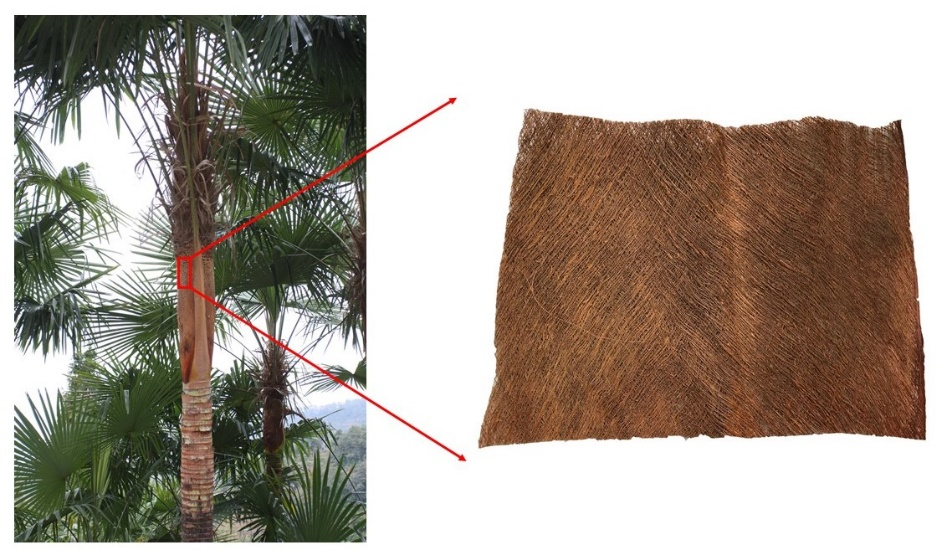Fig. 1. The palm trees and palm fibers

The fractal theory describes the self-similar structures, which can be presented at multiple scales within a given structure (Rian and Asayama 2016). Mandelbrot first applied fractal concepts to describe complex shapes and patterns via fractal dimensions (Losa 2016). Previous studies have been conducted on characterizing a materials’ structure and properties by fractal theory. For instance, Fan et al. (2006) established the relationship between the fractal dimensions and porosity of the wood, which has been used to predict the effective thermal conductivity perpendicular to the fibers. Other interesting studies considered natural composites’ macrostructure. For example, the porous structure of lignocellulose could be characterized by the fractal dimension methodology (Chi et al. 2017). However, the multi-scale microscopic and mechanical properties have rarely been studied by the fractal theory. As mentioned earlier, the cross-sectional microstructure of palm fiber may illustrate a multi-scale self-similar hierarchical organization, which means that a relationship between the structure and mechanical properties of palm fibers can be established by using the fractal theory.

To optimize biomimetic materials and composite structural design and composite fabrication, as well as expand the application of palm ﬁbers in civil industries, understanding the structure/property relationship is of great importance. In this work, a three-circle fractal theoretical model was established, and the palm fiber’s tensile properties on different scales were studied. Characters of stress, fracture energy, and Young’s modulus on different scales were calculated and verified by tensile testing and atomic force microscope (AFM).

EXPERIMENTAL

Fractal model

As discussed earlier, palm fiber is a multi-cell fiber. The multi-cell structure endows palm fiber with porous properties. Figure 2 shows the palm fibers cross-sectional structure. The palm fiber is tightly linked with several individual hollow cells. This multicellular structure endows the palm fiber with a unidirectional, regular, and hollow fabrication, which allows palm fibers to possess unique self-similar characteristics.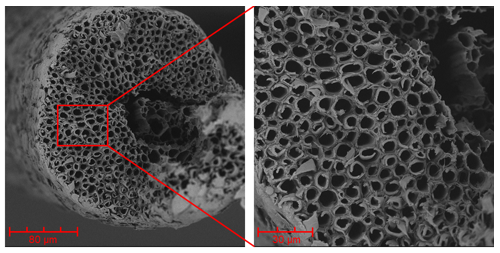Fig. 2. SEM images of palm fiber cross-section

Based on previous studies, the author has sketched the hierarchical organization of palm fiber from microcosmic to macroscopical scales. Figure 3 presents the hierarchical assembly of the palm fiber, i.e., cellulose molecular chains (f), fibril filaments (e), microfibrils (d), and plant cells (b). Moreover, the plant cell (c) consists of the lumen, middle lamella, and primary and secondary walls, as shown in Fig. 3. The plant cells grow parallel to the longitudinal direction and are closely arranged in the transverse direction. The cells (b) formed a palm fiber (a) with a rough surface and randomly distributed scale-like material (Zhang et al. 2015). Palm fiber’s hierarchical organization allows for optimization to achieve mechanical efficiency, and the hierarchical organization on different scales may contribute to different tensile properties of the palm fibers (Wegst 2011).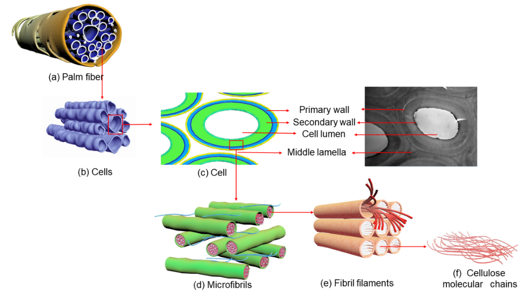Fig. 3. Hierarchical assembly of palm fiber: (a) Palm fiber, (b) Cells, (c) Cell, (d) Microfibrils, (e) Fibril filaments, and (f) Cellulose molecular chains

Most organic materials exhibit a fractal-like organization and self-similarity hierarchical structure at the microscopic level (Reznikov et al. 2018). According to the three-point Cantor set, as proposed by a German mathematician, G. Cantor (Bossard et al. 2016), a three-circle fractal set was built for the multi-scale layer of the palm fiber’s ultrastructure with three-circle fiber-reinforced beams. As shown in Fig. 4, palm fiber can be regarded as a homogeneous cylinder composed of multiple cells. The authors took three cells in the homogeneous cylinder as one cell fiber unit to represent the overall characteristics of the fiber. The multi-scale fractal hierarchical structure of palm fiber includes, from large to small, palm fiber, cells, microfibrils, fibril filaments, and cellulose molecular chains. The multi-scale three-circle fractal model describes four hierarchical assemblies: cellulose molecular chain to fibril filament (CF), fibril filament to microfibril (FM), microfibril to cell (MC), and cell to palm fiber (CP). In tensile testing, the author took palm cells as homogeneous fibers to calculate their mechanical characters. Therefore, the simulating model was also presented as a homogeneous unit at the scale of microfibril to cell (MC).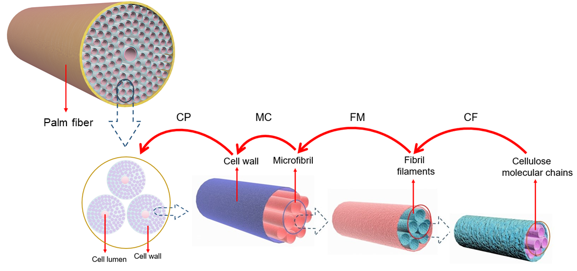Fig. 4. Fractal model of palm fiber

In the model, each transverse section has a unique, non-integer fractal dimension at different hierarchical organization levels. The relationship between fractal dimensions and physical quantity is used to characterize the mechanical properties of the fiber, and the fractal dimensions of different materials were non-uniform in both vertical and horizontal directions. For instance, individual fibrin fiber has a fractal dimension of 1.3 (Guthold et al. 2004) along the cross-section and the wool has a three-circle fractal dimension of 1.4311 (Li et al. 2013) in the transverse macroscopic direction. In this work, the microscopic fractal dimension was calculated using the theoretical model, and the cell fractal dimension was calculated using the pore fractal method (Tang et al. 2015). The mixture principle method (Vilaseca et al. 2010) was used to calculate the physical quantity of the cell wall, which is mainly composed of cellulose, hemicellulose, and lignin.

Theoretically, hemicellulose and lignin are the matrix materials of the palm fiber cell wall, rendering stable properties, whereas cellulose is the skeleton material (Jin et al. 2015). Herein, the matrix materials, hemicellulose and lignin, were defined as the soft phases, whereas the skeleton material, cellulose, was defined as the hard phase. Based on the mixture principle, stress can be expressed as follows (Li et al. 2013),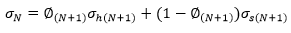(1)

where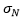refers to the total stress in (N + 1) level,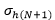represents the stress of the hard phase in (+ 1) level,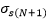corresponds to the stress of the soft phase in (+ 1) level, and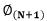denotes the total volume fraction of the hard phase in (+ 1) level, which can be expressed as,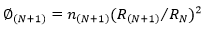(2)

where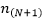refers to the number of components in (N + 1) level,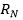corresponds to the radius of the model at N level, and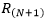represents the radius of the model at (N + 1) level.

By substituting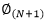into Eq. 1, the following equation can be obtained: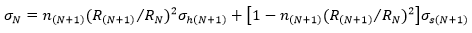(3)

The concentration force in level of the hard phase can be defined as: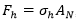, and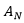is the area in N level. The number of hard phases in N level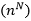should be known to calculate the area of the hard phase in N level. Thus, the area in N level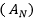becomes equal to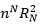. From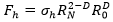(Pugno and Carpinteri 2008) and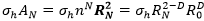, the derivation gives Eq. 4,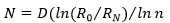(4)

where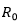refers to the diameter (nm or µm) of fibers and D represents the fractal dimension. By substituting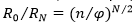, where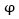refers to the volumetric fraction of each level, as derived by Pugno and Carpinteri (2008) into Eq. 4, the fractal dimension can be obtained as follows: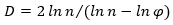(5)

From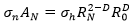and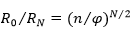, the total volume fraction of the hard phase can be given as: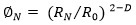(6)

By substituting Eq. 5 into Eq. 1, the following stress formula can be obtained (Li et al. 2013):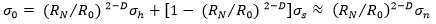(7)

Similarly, by substituting the fracture energy and Young’s modulus into Eq. 1, the following equations can be obtained: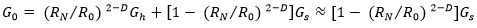(8)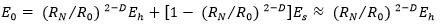(9)

In biomaterials, the stress and Young’s modulus of the hard phase were far higher than the soft phase, whereas the fracture energy of the hard phase was much smaller than the soft phase.

Apparatus and Methodology

Palm fibers were obtained from Yunnan province, China. The surface morphology of palm fibers and cells were determined from the scanning electron microscope (Phenom World, Eindhoven, Netherlands) under a high vacuum. Dry samples were mounted on aluminum stubs and coated with gold (Cressington Scientific Instruments Ltd., Watford, UK) to make the surfaces conductive. Imaging was then performed at beam accelerating voltages of 10 kV. The transverse sections were observed under the optical microscope and photographed according to the paraffin section method. The diameter and fractal dimension of palm fibers were determined by the photographs of the transverse section. ImageJ software (National Institutes of Health, v.1.52a, Bethesda, MD, USA) was used to obtain the average diameter by calculating photographic measurements three times. The transverse fractal dimensions of the palm fiber were calculated from the photographs by using the MATLAB box-counting program (MathWorks, v.R2016a, Natick, MA, USA).

The cell transverse section images of the palm fiber were obtained using the transmission electron microscope. The fractal dimensions of the cell transverse section were also calculated using the MATLAB box-counting program. No other treatment was performed on the fibers prior to testing, except for the removal of dust from the surface. The tensile test of palm fiber was conducted using the MTS E44 universal testing machine (MST Industrial Systems (China) Co., Ltd., Shanghai, China), with a gauge length (GL) of 10, 20, 30, and 40 mm and displacement speed of 2 mm/min. At least 30 samples were tested at each GL. This displacement rate corresponds to a nominal strain rate range of approximately from 2.083 × 10 – 4/s (GL = 40 mm) to 8.333 × 10 – 4/s (GL = 10 mm) in the linear region of the stress–strain curve.

Palm fiber cells were chemically isolated from the plant material in a solution comprised hydrogen peroxide and glacial acetic acid. The cells were connected to carbon fiber with bridging technology and the bridge can solidify at 60°C using epoxy for 24 hours, followed by an additional balance at room conditions for 24 h. It was ensured that the epoxy is cured before stretching. The tensile test of palm cell was performed by using YG004 single fiber strength tester (Dahua Electronic Instruments, Changzhou, China), with a displacement speed of 1 mm/min.

Atomic force microscopy (Dimension Icon, Bruker, West Grove, PA, USA) was employed to further understand the compression behavior of the transverse section of palm fibers. Atomic force microscopy measurements were conducted at room temperature under atmospheric air. The Young’s modulus was determined by using PeakForce QNM (Quantitative NanoMechanics, Denver, CO, USA). Topography and QNM were simultaneously measured at 256 × 256 pixels/scan, which resulted in force curves. The Young’s modulus was derived from the force curves according to the Derjaguin-Muller-Toropov (DMT) model.

RESULTS AND DISCUSSION

Fractal Dimension

The fractal dimensions of fibers were different at each hierarchical organization level. The diameter of cellulose molecular chain, fibril filament, and microfibril were approximately 0.6 nm, 3.5 nm, and 10 to 12 nm, respectively (Huang et al. 2003). By assuming that there were 40 cellulose molecular chains in the fibril filament, the radius at the microscopic level of the cellulose molecular chain (r) and the fibril filament (R) can be estimated as 0.3 nm and 1.75 nm, respectively. The percentage of cellulose chains in the fibril filament cross-section becomes equal to M= 40 pi 0.3/ (pi1.752) = 1.1755 Therefore, the fractal dimension of fibril filaments (Kerr and Goring 1975) can be expressed as D= log117.55 / log100 = 1.0361. The above-mentioned method was further applied to calculate the fractal dimension of microfibrils by assuming that a microfibril has a radius of 5.5 nm and is composed of three fibril filaments. The results reveal that the microfibril cross-section has the percentage of fibril filament (M2) of 0.30372 and the fractal dimension (D2) of 0.74124, as shown in Table 1.

Table 1. Diameters and Fractal Dimensions of Component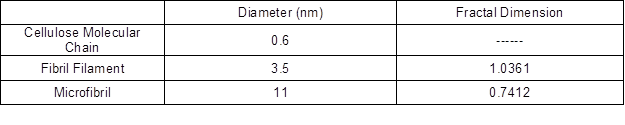The diameters and fractal dimensions of the palm fiber and cell are presented in Fig. 5. The diameter of palm fibers ranged from 200 to 1400 μm, whereas the diameter of palm cells ranged from 8 to 14 μm. The average diameter of palm fibers is 565 μm, while that for palm cell is 11.4 μm. The fractal dimensions of the transverse section of both palm fiber and the palm cell ranged from 1.7 to 2.0. The average fractal dimension of palm fibers is 1.97, while that for palm cell is 1.88. However, the fractal dimension of microfibers was smaller than the fibril filaments and palm cells, which can be ascribed to the relatively lower content of cellulose (~28.2%) (Zhang et al. 2015) and a large amount of interstitial structure in the cell wall. For palm fibers and cells, there is no one-to-one relationship between the diameter and fractal dimension. This may be because of the randomness of the size of cell cavity and thickness of the intercellular substance in natural fibers. It is this unique structure that makes palm fibers and the cells exhibit fractal properties.

Mechanical Properties

The experimental results of tensile stress, fracture energy, and Young’s modulus of the cell and palm fiber are shown in Fig. 6. As mentioned above, palm fiber is a multi-cell natural fiber at the palm leaf sheath. Palm fibers are composed of numerous elongated individual hollow cells that are tightly connected. The palm fiber cells are single fibers that are separated after stripping palm fibers. The cells tested exhibited a quasi-linear stress-strain behavior to failure, which is different from that of palm fibers. Palm fibers exhibited typical stress-strain failure behavior curves of natural composites. They were composed of an initial linear region and followed the non-linear portion. After the yield point was reached, plastic deformation occurred until breakage. The average values of stress, fracture energy, and Young’s modulus of the cell were 219.2 MPa, 36.3 J/m3, and 648.2 MPa, whereas for the palm fibers were 145.9 MPa, 18.1 J/m3, and 607.3 MPa, respectively. However, the average elongation at break of cell and palm fiber was 30.8% and 35.1%, respectively.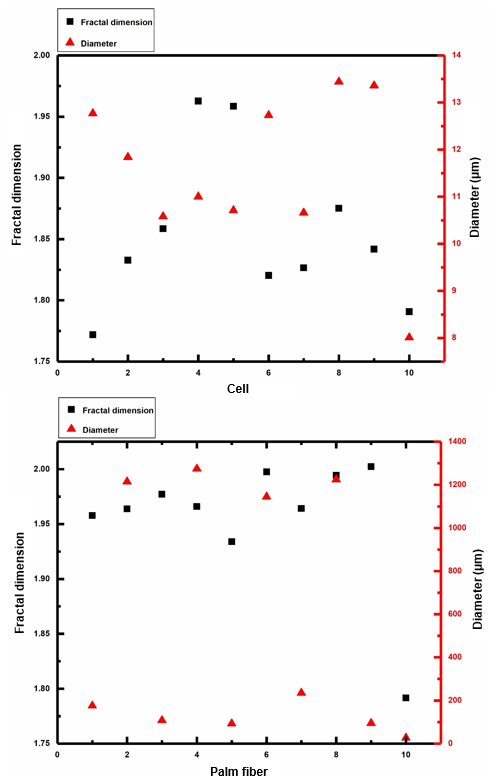Fig. 5. Diameters and fractal dimensions of the cell and palm fiber

Palm cell is composed of a cell cavity and cell wall. The cell wall main components are lignin, cellulose, and hemicellulose. The components are closely connected to form a multilayer structure of the cell wall. This kind of cell wall is not easy to deform when stretched, showing high stress, breaking energy, Young’s modulus, and low elongation at break. Palm fiber is a multicellular fiber, which is composed of matrix and cells. Due to the relatively weak intercellular and matrix connection, palm fibers firstly exhibit intercellular slippage and delamination during the stretching process. These mechanical behaviors of palm fibers cause the lower stress, fracture energy, Young’s modulus, and the higher elongation at break (Zhang et al. 2015).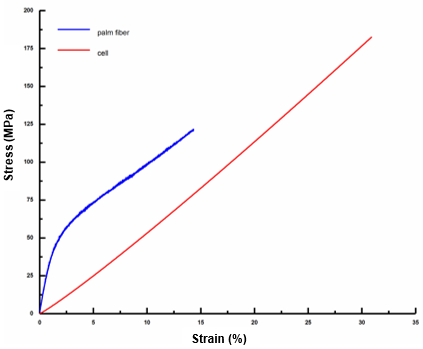Fig. 6. Stress-strain curve of the fiber’s cell and palm fiber

To further verify the accuracy of the cell stretching curve and reliability of the model calculation parameter settings, the authors conducted AFM experiments on the cell wall cross-section. Figure 7 presents the DMT modulus of the cell, which was computed from the AFM images. It can be readily observed that the Young’s modulus of the cells exhibited a significant variation, ranging from 104.7 to 639.6 MPa, which is indicated by the color change in the AFM image.

The darker color indicates a small value of Young’s modulus, whereas the light color represents a higher value of Young’s modulus. The experimentally measured Young’s modulus of the cell was 648.2 MPa, which is consistent with the AFM results and indicates the reliability of the experiment data. In fact, the dark color represents the soft phase with higher Young’s modulus, whereas light color represents a hard phase with lower Young’s modulus. These observations were also consistent with the three-circle fractal model.